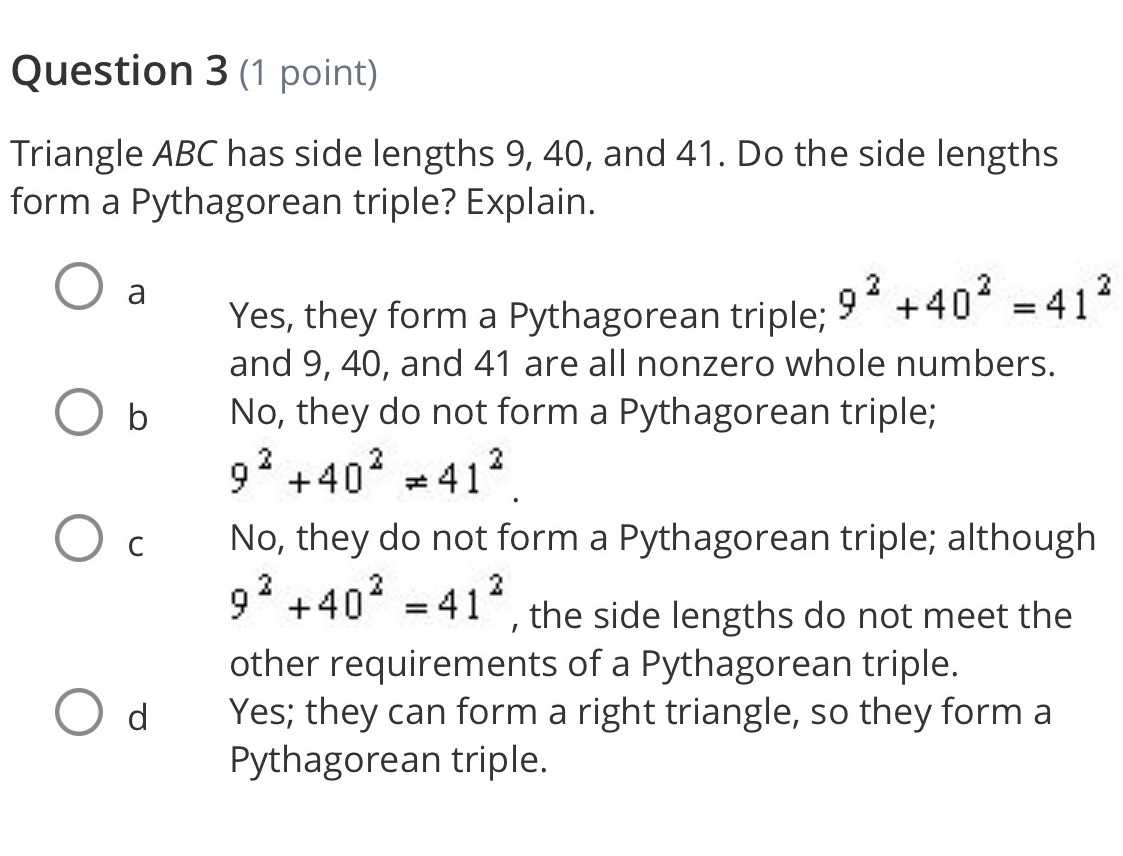### Still have math questions?

Trigonometry
QuestionTriangle $$A B C$$ has side lengths $$9,40 ,$$ and $$41 .$$ Do the side lengths form a Pythagorean triple? Explain.

a Yes, they form a Pythagorean triple; $$9 ^ { 2 } + 40 ^ { 2 } = 41 ^ { 2 }$$ and $$9,40$$ , and $$41$$ are all nonzero whole numbers.

b No, they do not form a Pythagorean triple; $$9 ^ { 2 } + 40 ^ { 2 } \neq 41 ^ { 2 }$$ .

c No, they do not form a Pythagorean triple; although $$9 ^ { 2 } + 40 ^ { 2 } = 41 ^ { 2 }$$ , the side lengths do not meet the other requirements of a Pythagorean triple.

d.Yes; they can form a right triangle, so they form a Pythagorean triple.

Pythagorean triple

$$largestside^ 2 = shortestside^ 2+ secondshortestside^ 2$$

given sides 9,40,41

$$41^ 2 = 1681\\ 40^ 2= 1600\\ 9^ 2= 81\\ 41^ 2= 40^ 2+ 9^ 2\\ so they are pytagorean triple$$